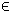## 22LINRAT Problem

The linearly constrained linear ratio (LINRAT) problem is defined as

 min x
c1 x
c2 x
subject to xL x xU
bL Ax bU
(24)
where c1,c2,x,xL,xURn, bL,bURm2, and ARm1 × n.

The LINRAT solution can be obtained by the use of any suitable linear TOMLAB solver.

The following file illustrates how to solve a LINRAT problem in TOMLAB.

File: tomlab/quickguide/linratQG.m

Open the file for viewing, and execute linratQG in Matlab.
```% linratQG is a small example problem for defining and solving
% linear ratio programming problems using the TOMLAB format.

Name = 'Linear Ratio 1';
x_0  = [2;2;2;2];          % Initial value
x_L  = [1;2;3;4];          % Lower bounds on x
x_U  = [100;100;50;50];    % Upper bounds on x

% Define the numerator and denominator for the objective
c1 = [3;7;9;11];
c2 = [20;15;10;5];

% Add the linear constraint x(1) + x(2) + x(3) + x(4) - 20 <= 0
% Write the constraint as x(1) + x(2) + x(3) + x(4) <= 20

% The A matrix could be specified dense or sparse
% A   = sparse([1 1 1 1]);

A   = [1 1 1 1];
b_L = -inf;
b_U = 20;

c = zeros(4,1); % Dummy objective

% Generate an LP problem using the Tomlab Quick format
% Use mipAssign if solving a mixed-integer problem
Prob = lpAssign(c, A, b_L, b_U, x_L, x_U, x_0, Name);
Prob.QP.c1 = c1;
Prob.QP.c2 = c2;

Prob.SolverRat = 'minos';

% One may set other solvers:
% Prob.SolverRat = 'cplex';
% Prob.SolverRat = 'xa';
% Prob.SolverRat = 'snopt';
% Prob.SolverRat = 'milpSolve';

% Set print level 1 to get output from PrintResult at the end
PriLev = 1;
Prob.PriLevOpt = 0;

Result  = tomRun('linRatSolve', Prob, PriLev);```